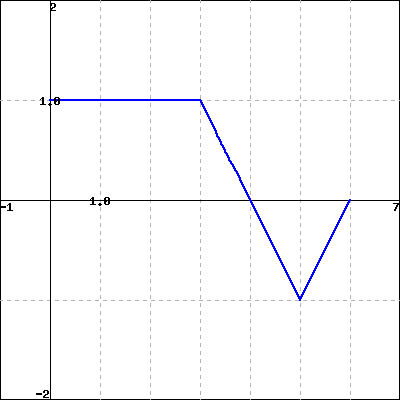The figure below shows $f$.(Click on the graph for a larger version.)

If $F'=f$ and $F(0)=0$, find $F(b)$ for $b=$1, 2, 3, 4, 5, 6, and fill these values in the following table.

 $b$ 1 2 3 4 5 6 $F(b)$